# JP5419825B2 - Signal processing system and signal processing method - Google Patents

## Info

Publication number
JP5419825B2
JP5419825B2 JP2010180257A JP2010180257A JP5419825B2 JP 5419825 B2 JP5419825 B2 JP 5419825B2 JP 2010180257 A JP2010180257 A JP 2010180257A JP 2010180257 A JP2010180257 A JP 2010180257A JP 5419825 B2 JP5419825 B2 JP 5419825B2
Authority
JP
Japan
Prior art keywords
matrix
measurement matrix
weighting
unit
random
Prior art date
Legal status (The legal status is an assumption and is not a legal conclusion. Google has not performed a legal analysis and makes no representation as to the accuracy of the status listed.)
Active
Application number
JP2010180257A
Other languages
Japanese (ja)
Other versions
JP2011193431A (en
Inventor

Original Assignee

Priority date (The priority date is an assumption and is not a legal conclusion. Google has not performed a legal analysis and makes no representation as to the accuracy of the date listed.)
Filing date
Publication date
Priority to JP2010036421 priority Critical
Priority to JP2010036421 priority
Application filed by 日本電信電話株式会社 filed Critical 日本電信電話株式会社
Priority to JP2010180257A priority patent/JP5419825B2/en
Publication of JP2011193431A publication Critical patent/JP2011193431A/en
Application granted granted Critical
Publication of JP5419825B2 publication Critical patent/JP5419825B2/en
Application status is Active legal-status Critical
Anticipated expiration legal-status Critical

## Images

•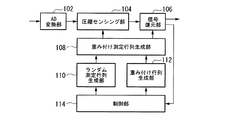•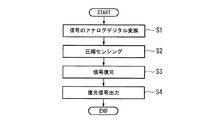•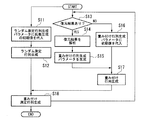••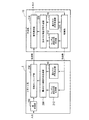•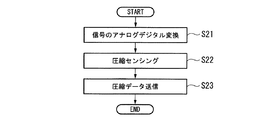•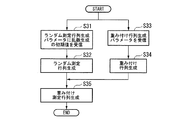•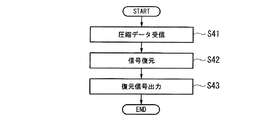•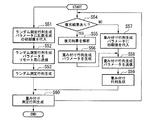•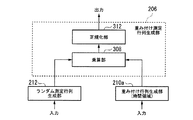•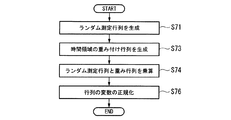•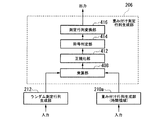••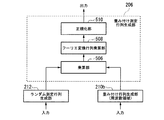•••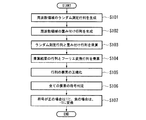•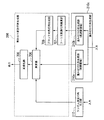•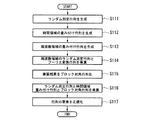•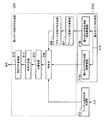••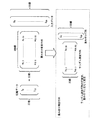••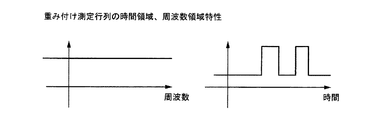••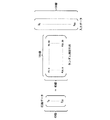•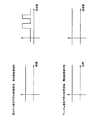•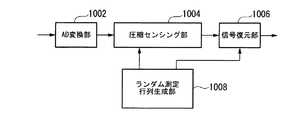•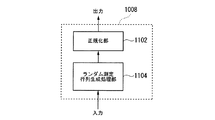••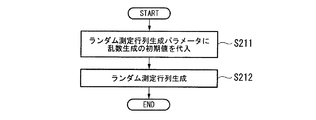## Description

The present invention relates to a signal processing system and a signal processing method for generating a weighted measurement matrix in compressed sensing for AD conversion, compression, and restoration of a signal.

Conventionally, it is known that a network band can be effectively utilized by AD-converting, compressing, and transmitting a signal (data series). In this specification, a technique for AD-converting, compressing, and restoring a signal is referred to as a compressed sensing technique. In the conventional compressed sensing technology, a signal is multiplied by a random measurement matrix to generate compressed data. This random measurement matrix is composed of random Gaussian variables, and for the number of input data (signal), The number of rows is such that the number of output data (compressed data) becomes small.

The concept of conventional compression sensing is shown in FIG. 26 (for example, see Non-Patent Documents 1 and 2). FIG. 26 assumes a sparse signal in the frequency domain or time domain. Since the signal is sparse in frequency or time, it is converted by a random measurement matrix composed of 40 × 100, and 40 compressed data are obtained. As shown in FIG. 27, the characteristics of the time domain and the frequency domain of the random measurement matrix are constant without changing with respect to the time axis and the frequency axis.

A system configuration for performing conventional compressed sensing is shown in FIG. In FIG. 28, the input signal is converted from an analog signal to a digital signal by the AD conversion unit 1002, and the conversion result is output to the compression sensing unit 1004. The random measurement matrix generation unit 1008 generates a random measurement matrix composed of random Gaussian variables. The compression sensing unit 1004 compresses the input digital signal using the random measurement matrix generated by the random measurement matrix generation unit 1008. The compression result is output to the signal restoration unit 1006. The signal restoration unit 1006 restores the input compressed data using a restoration algorithm such as a random measurement matrix and an L1-minimization restoration algorithm (see, for example, Non-Patent Document 3), and outputs the restored signal.

FIG. 29 shows a configuration of a conventional random measurement matrix generation unit 1008. In FIG. 29, a random measurement matrix generation processing unit 1104 receives a parameter (seed) for generating a random Gaussian variable, and generates a random measurement matrix. The normalization unit 1102 normalizes the random measurement matrix and outputs the normalized random measurement matrix. FIG. 30 shows a flowchart of processing operations of conventional compression sensing. First, analog-digital conversion of the received signal is performed (step S201), and the received signal is compressed using a random measurement matrix (step S202). The compressed data is restored using the random measurement matrix used at the time of compression and the L1-minimization restoration algorithm (step S203) and output (step S204). FIG. 31 shows a flowchart of the random measurement matrix generation processing operation. First, an initial value (seed) for generating a random Gaussian variable is substituted into a random measurement matrix generation parameter (step S211), and a random measurement matrix is generated using the random measurement matrix generation parameter (step S212).

E. Candes et al., "An Introduction to Compressive Sampling," IEEE Signal Processing Magazine, pp. 21-30, March, 2008. E. Candes et al., "Near-Optimal Signal Recovery from Random Projections: Universal Encoding Strategies?" IEEE Transaction on Information Theory, pp. 5406-5425, December, 2006. E. Candes et al., "L1-Magic: Recovery of Sparse Signals via Convex Programming," Oct. 2005., http: // www.acm.caltech.edu/l1magic/downloads/l1magic.pdf

By the way, in the conventional compressed sensing technology, even when the signal has a high density region and a low density region in frequency density or time density (sparse), the weights of the variables of the random measurement matrix are uniformly equal, and the specific frequency Or do not weight for a specific time. In other words, because compression sensing is performed using the same random measurement matrix as a high-density area with a large amount of information even for a low-density area with a small amount of information, the low-density area with a small amount of information becomes overhead and compression efficiency does not improve. There's a problem.

The present invention has been made in view of such circumstances, and in compression sensing for AD conversion, compression, and restoration of a signal, signal processing that can improve compression efficiency by optimizing the generation of a weighted measurement matrix. It is an object to provide a system and a signal processing method.

The present invention is a signal processing system that compresses and decompresses a signal having a sparse property in the frequency domain or in the time domain, and converts an input analog signal into a digital signal and outputs the digital signal, A control unit that generates a random measurement matrix generation parameter for generating a random measurement matrix and generates a weighting matrix generation parameter for generating a weighting matrix based on an analysis result of the restoration result; and the random measurement matrix generation parameter A random measurement matrix generation unit that generates the random measurement matrix based on the weighting matrix generation unit that generates the weighting matrix based on the weighting matrix generation parameter, and generates a weighted measurement matrix using the weighting matrix and the random measurement matrix. A weighting measurement matrix generation unit that performs the A measurement using the weighting measurement matrix. A compression sensing unit for compressing the digital signal output from the conversion unit; and restoring the digital signal by using the weighted measurement matrix for the compressed digital signal; And a signal restoration unit for notifying the control unit.

The present invention is a signal processing system composed of a central station and a remote station in order to compress and decompress a signal having a sparse property in the frequency domain or in the time domain. An analog-to-digital converter that converts the analog signal into a digital signal for output, a random measurement matrix generation unit that generates a random measurement matrix based on a random measurement matrix generation parameter, and a weighting matrix that generates a weighting matrix based on the weighting matrix generation parameter A generating unit; a weighting measurement matrix generating unit that generates a weighting measurement matrix using the weighting matrix and the random measurement matrix; and a compression that compresses the digital signal output from the AD conversion unit using the weighting measurement matrix A communication unit that performs communication between the sensing unit and the central station; A control unit for generating the random measurement matrix generation parameter for generating a measurement matrix and generating the weighting matrix generation parameter for generating the weighting matrix based on the analysis result of the restoration result, and the random measurement matrix generation A random measurement matrix generation unit that generates the random measurement matrix based on a parameter; a weighting matrix generation unit that generates the weighting matrix based on the weighting matrix generation parameter; and a weighting measurement matrix using the weighting matrix and the random measurement matrix A weighted measurement matrix generating unit for generating the signal, and a signal for performing restoration of the digital signal using the weighted measurement matrix for the signal compressed by the compression sensing unit, and notifying the control unit of the output of the restoration result and the restoration result Communication for performing communication between the restoration unit and the remote station Characterized by including the.

In the present invention, the weighting matrix generation unit generates the weighting matrix in the time domain, and the weighting measurement matrix generation unit multiplies the random measurement matrix and the weighting matrix to obtain the weighting measurement matrix; And a normalization unit for normalizing the weighting measurement matrix.

In the present invention, the weighting matrix generation unit generates the weighting matrix in the frequency domain, and the weighting measurement matrix generation unit multiplies the random measurement matrix and the weighting matrix to obtain the weighting measurement matrix; And a Fourier transform matrix multiplier for multiplying the weighted measurement matrix by a Fourier transform matrix such as FFT, and a normalizer for normalizing the weighted measurement matrix.

In the present invention, the random measurement matrix generation unit generates the random measurement matrix, the weighting matrix generation unit generates the weighting matrix in the time domain and the weighting matrix in the frequency domain, and the weighting measurement matrix generation unit Includes a Fourier transform matrix multiplier that multiplies the weighting matrix in the frequency domain and a Fourier transform matrix such as FFT, a block diagonal matrix generator that blocks the output of the Fourier transform matrix multiplier, and the random matrix A multiplication unit that multiplies the measurement matrix, the weighting matrix in the time domain, and the matrix output from the block diagonal matrix generation unit to obtain the weighting measurement matrix; and a normalization unit that normalizes the weighting measurement matrix It is characterized by doing.

In the present invention, the weighting measurement matrix generation unit is configured to determine a sign of each variable of the weighting measurement matrix output from the normalization unit, and the weighting measurement based on a determination result of the coding determination unit. It further includes a measurement matrix conversion unit that converts each variable of the matrix into “1” or “−1” according to its sign.

The present invention provides an AD conversion unit, a control unit, a random measurement matrix generation unit, a weighting matrix generation unit, a compression unit, in order to perform compression and decompression of a signal having a sparse property in the frequency domain or the time domain A signal processing method in a signal processing system including a sensing unit and a signal restoration unit, wherein the AD conversion unit converts an input analog signal into a digital signal and outputs the digital signal, and the control unit includes: A control step for generating a random measurement matrix generation parameter for generating a random measurement matrix, generating a weighting matrix generation parameter for generating a weighting matrix based on an analysis result of the restoration result, and the random measurement matrix generation unit, A random measurement matrix generating step for generating the random measurement matrix based on the random measurement matrix generation parameter; The weighting matrix generation unit generates the weighting matrix based on the weighting matrix generation parameter, and the weighting measurement matrix generation unit generates the weighting measurement matrix using the weighting matrix and the random measurement matrix. A weighted measurement matrix generating step, a compression sensing step in which the compressed sensing unit compresses the digital signal output from the AD conversion unit using the weighted measurement matrix, and the signal restoration unit is compressed. The digital signal is restored using the weighted measurement matrix, and a restoration result is output and a signal restoration step of notifying the controller of the restoration result is provided.

The present invention provides an AD conversion unit, a random measurement matrix generation unit, a weighting matrix generation unit, and a weighted measurement matrix generation in order to compress and decompress a signal having a sparse property in the frequency domain or in the time domain A remote station including a communication unit that communicates with a central processing unit, a compressed sensing unit, and a central station, a control unit, a random measurement matrix generation unit, a weighting matrix generation unit, a weighting measurement matrix generation unit, A signal processing method in a signal processing system including a signal restoration unit and a central station including a communication unit that performs communication with the remote station, wherein the remote station receives an input from the AD conversion unit AD conversion step for converting the converted analog signal into a digital signal and outputting it, and the random measurement matrix generation unit generates a random measurement matrix based on a random measurement matrix generation parameter A random measurement matrix generation step, a weighting matrix generation step in which the weighting matrix generation unit generates a weighting matrix based on a weighting matrix generation parameter, and the weighting measurement matrix generation unit uses the weighting matrix and the random measurement matrix. A weighted measurement matrix generating step for generating a weighted measurement matrix, a compressed sensing step for compressing the digital signal output from the AD conversion unit using the weighted measurement matrix, and the communication unit Communication step of performing communication with the central station, and the central station generates the random measurement matrix generation parameter for the control unit to generate the random measurement matrix, and a restoration result Generation of the weighting matrix for generating the weighting matrix based on the analysis result of A control step for generating parameters, a random measurement matrix generation step for generating the random measurement matrix based on the random measurement matrix generation parameter, and a weighting matrix generation unit for generating the weighting matrix A weighting matrix generating step for generating the weighting matrix based on a parameter; a weighting measurement matrix generating step in which the weighting measurement matrix generating unit generates a weighting measurement matrix using the weighting matrix and the random measurement matrix; and the signal restoration A signal restoration step of performing restoration of the digital signal on the signal compressed by the compression sensing unit using the weighted measurement matrix, and notifying the control unit of an output of the restoration result and the restoration result; and the communication unit A communication step of performing communication with the remote station It is characterized by having.

The weighting matrix generation step generates the weighting matrix in the time domain, and the weighting measurement matrix generation step multiplies the random measurement matrix and the weighting matrix to obtain the weighting measurement matrix. And a normalizing step for normalizing the weighted measurement matrix.

In the present invention, the weighting matrix generation step generates the weighting matrix in a frequency domain, and the weighting measurement matrix generation step multiplies the random measurement matrix and the weighting matrix to obtain the weighting measurement matrix. And multiplying the matrix output from the step by a Fourier transform matrix such as FFT, and a normalizing step for normalizing the weighted measurement matrix.

In the present invention, the random measurement matrix generation step generates the random measurement matrix, and the weighting matrix generation step generates the weighting matrix in the time domain and the weighting matrix in the frequency domain, and the weighting measurement matrix generation step Multiplying the frequency domain weighting matrix by a Fourier transform matrix such as FFT, generating a block diagonal matrix by converting the output of the Fourier transform matrix multiplier into a block diagonal matrix, and the random measurement matrix A multiplication step for multiplying the weighting matrix in the time domain by the block diagonal matrix to obtain the weighting measurement matrix, and a normalization step for normalizing the weighting measurement matrix.

According to the present invention, the weighting measurement matrix generation step includes a code determination step for determining a sign of each variable of the weighting measurement matrix output by the normalization step, and the weighting based on a determination result of the encoding determination step. The method further includes a measurement matrix conversion step of converting each variable of the measurement matrix into “1” or “−1” according to the sign thereof.

According to the present invention, in compressed sensing, compression is performed by using known information such as reception timing of a received signal, frequency component of the received signal, frequency priority, time component, time priority, and reception level of the received signal. At the time of sensing, it is possible to weight a specific position in the frequency domain or weight a specific position in the time-frequency domain. Thereby, priority can be set in the frequency domain and the time domain, and flexible compression control according to the importance of the system and the channel state can be performed. In addition, the weighted random measurement matrix is not weighted because the ratio of the specific frequency and time included in the signal component is higher than the unweighted frequency and time. Compared to the random measurement matrix, the number of rows can be reduced, and the amount of calculation when performing compression sampling can be reduced. Further, since the number of rows of the random measurement matrix is proportional to the compression ratio of the signal, an effect of lowering the compression ratio (high compression) can be obtained as compared with the conventional case where no weight is given to the random measurement matrix. Further, since normalization is performed for each row, PAPR (Peak to Average Power Ratio) can be suppressed.

It is a block diagram which shows the structure of the signal processing system by the 1st Embodiment of this invention. 2 is a flowchart illustrating operations for performing data compression and decompression in the signal processing system illustrated in FIG. 1. 2 is a flowchart showing an operation of generating a weighted measurement matrix in the signal processing system shown in FIG. It is a block diagram which shows the structure of the communication system comprised from the one or more remote stations 1-N and the central station 10 in 2nd Embodiment, and the remote stations 1-N and the central station 10 are connected by the transmission line. . It is a block diagram which shows the structure of the remote station 1 and the central station 10 shown in FIG. It is a flowchart which shows operation | movement of the remote station 1 shown in FIG. It is a flowchart which shows the operation | movement which the remote station 1 shown in FIG. 5 produces | generates a weighting measurement matrix. It is a flowchart which shows operation | movement of the central office 10 shown in FIG. It is a flowchart which shows the operation | movement which the central station 10 shown in FIG. 5 produces | generates a weighting measurement matrix. It is a block diagram which shows the structure of the weighting measurement matrix production | generation part 206 in 3rd Embodiment. It is a flowchart which shows operation | movement of the weighting measurement matrix production | generation part 206 shown in FIG. 10, the random measurement matrix production | generation part 212, and the weighting matrix production | generation part 210a. It is a block diagram which shows the structure of the weighting measurement matrix production | generation part 206 in 4th Embodiment. 13 is a flowchart showing operations of a weighted measurement matrix generation unit 206, a random measurement matrix generation unit 212a, and a weighting matrix generation unit 210a shown in FIG. It is a block diagram which shows the structure of the weighting measurement matrix production | generation part 206 in 5th Embodiment. It is a flowchart which shows operation | movement of the weighting measurement matrix production | generation part 206 shown in FIG. 14, the random measurement matrix production | generation part 212, and the weighting matrix production | generation part 210b. It is a block diagram which shows the structure of the weighting measurement matrix production | generation part 206 in 6th Embodiment. 17 is a flowchart illustrating operations of the weighted measurement matrix generation unit 206, the random measurement matrix generation unit 212, and the weighting matrix generation unit 210b illustrated in FIG. It is a block diagram which shows the structure of the weighting measurement matrix production | generation part 206 in 7th Embodiment. It is a flowchart which shows operation | movement of the weighting measurement matrix production | generation part 206 shown in FIG. 18, the random measurement matrix production | generation part 212, and the weighting matrix production | generation part 210c. It is a block diagram which shows the structure of the weighting measurement matrix production | generation part 206 in 8th Embodiment. 21 is a flowchart showing operations of a weighted measurement matrix generation unit 206, a random measurement matrix generation unit 212, and a weighting matrix generation unit 210c shown in FIG. It is explanatory drawing which shows the concept of the weighted compression sensing method of this invention. It is explanatory drawing which shows the example of operation | movement of a block diagonal matrix production | generation part. It is explanatory drawing which shows the characteristic of the time domain of a weighting measurement matrix and a random measurement matrix, and a frequency domain. It is explanatory drawing which shows the characteristic of the time domain of a weighting measurement matrix and a random measurement matrix, and a frequency domain. It is explanatory drawing which shows the concept of the conventional compression sensing. It is explanatory drawing which shows the characteristic of the time domain of a weighting measurement matrix and a random measurement matrix, and a frequency domain. It is a block diagram which shows the system configuration | structure which performs the conventional compression sensing. It is a block diagram which shows the structure of the conventional random measurement matrix production | generation part 1008. FIG. It is a flowchart which shows the processing operation of the conventional compression sensing. It is a flowchart which shows the conventional random measurement matrix production | generation process operation | movement.

Hereinafter, a signal processing system according to an embodiment of the present invention will be described with reference to the drawings. First, the weighted compression sensing method of the present invention will be described with reference to FIG. The input data is compressed by multiplying a sparse signal in the frequency domain or time domain by a weighted measurement matrix. In FIG. 22, 100 pieces of data are compressed into 20 pieces. The weighted measurement matrix is generated from a random measurement matrix generated by random Gaussian variables and a weighting matrix. Since the weighting matrix weights a specific frequency or time, the information amount of the weighted frequency or time can be made larger than the frequency or time that is not weighted. Thereby, since the number of simultaneous equations required when restoring the weighted frequency and time can be reduced, the number of rows of the random measurement matrix can be reduced. The number of rows in the weighted measurement matrix changes according to the weighting. FIG. 27 shows characteristics of the time domain and frequency domain of the weighted measurement matrix when weighting the frequency domain. Although the time domain characteristics of the weighted measurement matrix are constant regardless of time, the frequency domain characteristics are greatly emphasized in the frequency domain characteristics. FIG. 24 shows the characteristics of the time domain and frequency domain of the weighted measurement matrix when weighting the time domain. The frequency domain characteristic of the weighted measurement matrix is constant regardless of the frequency, but the time domain characteristic is greatly emphasized in the time domain characteristic. FIG. 25 shows the time domain and frequency domain characteristics of the weighted measurement matrix when weighting the time and frequency domains. The time domain and frequency domain characteristics of the weighted measurement matrix are greatly emphasized in the weighted time domain and frequency domain characteristics.

<First Embodiment>
Next, the configuration of the signal processing system according to the first embodiment of the present invention will be described with reference to FIG. FIG. 1 is a block diagram showing the configuration of the embodiment. In FIG. 1, an AD converter 102 receives a received signal, performs analog-digital conversion, and outputs digital data. The compression sensing unit 104 compresses the digital data using the weighted measurement matrix generated by the weighted measurement matrix generation unit 108 and outputs the compressed data. The signal restoration unit 106 receives the compressed data output from the compressed sensing unit 104 as an input, performs signal restoration using the same weighting measurement matrix as the weighting measurement matrix used when data compression is performed, and restores the restored data. Is output. At this time, a restoration algorithm such as L1-minimization operates. In addition, the restoration data is output to the control unit 114 as known information. The weighted measurement matrix generation unit 108 receives the random measurement matrix and the weighting matrix generated by the random measurement matrix generation unit 110 and the weighting matrix generation unit 112, respectively, and generates a weighted measurement matrix. The weighted measurement matrix generation unit 108 outputs the generated weighted measurement matrix to the compressed sensing unit 104 and the signal restoration unit 106, respectively.

The random measurement matrix generation unit 110 receives a random measurement matrix generation parameter output from the control unit 114 and generates a random measurement matrix. The generated random measurement matrix is output to the weighted measurement matrix generation unit 108. The weighting matrix generation unit 112 receives the weighting matrix generation parameter output from the control unit 114 and generates a weighting matrix. The generated weighting matrix is output to the weighted measurement matrix generating unit 108. The control unit 114 receives the restoration data output from the signal restoration unit 106. The input restoration data is treated as known information, weight generation parameters are generated based on the known information, and the number of rows of the random measurement matrix is determined. The information obtained from the known information is the signal generation period, the frequency at which the signal exists, the time at which the signal exists, and the reception level of the signal. The control unit 114 uses a random number initial value for generating a random Gaussian variable as a random measurement matrix generation parameter. The random measurement matrix generation parameter and the weighting matrix generation parameter are output to the random measurement matrix generation unit 110 and the weighting matrix generation unit 112, respectively.

Next, with reference to FIG. 2, the operation | movement which performs data compression and decompression | restoration in the signal processing system shown in FIG. 1 is demonstrated. FIG. 2 is a flowchart showing operations for performing data compression and decompression in the signal processing system shown in FIG. First, the AD converter 102 converts the input analog signal into a digital signal (step S1). The compression sensing unit 104 compresses this digital signal using a weighted measurement matrix (step S2). Subsequently, the signal restoration unit 106 restores the compressed data using a weighted measurement matrix obtained by compressing the compressed data and a restoration algorithm such as L1-minimization (step S3), and outputs the restored signal. (Step S4).

Next, an operation for generating a weighted measurement matrix in the signal processing system shown in FIG. 1 will be described with reference to FIG. FIG. 3 is a flowchart showing an operation of generating a weighted measurement matrix in the signal processing system shown in FIG. First, the control unit 114 sets an initial value of a random number for generating a random Gaussian variable for generating a random measurement matrix as a random measurement matrix generation parameter (step S11). The random measurement matrix generation unit 110 generates a random measurement matrix using the random measurement matrix generation parameter (step S12).

Next, the control unit 114 determines whether there is a restoration result (step S13). When the restoration result exists, the control unit 114 analyzes the restoration result, and receives the signal reception period, the frequency at which the signal exists, the time at which the signal exists, the reception level of the signal, or their reception level. Two or more combinations are obtained and used as known information (step S14). The control unit 114 determines the frequency and time for weighting based on the known information, and sets the weighting matrix generation parameter (step S15). In this method, a high weight is applied to a region where a signal component is large on the frequency or time axis, and a weight is reduced to a portion other than that. At this time, the number of rows of the random measurement matrix is also determined. As shown in FIG. 22, the principle of compression is that each of the compressed data (output signal) is output by applying a weight matrix to the transmission signal sequence (x0 to x99). Therefore, each of the output signals includes information on all input signals, and the more output signals there are, the higher the possibility that the signals can be restored. Here, if weighting is performed according to the characteristics of the input signal, restoration can be performed with only a smaller number of output signals, so that the number of rows of the random measurement matrix can be reduced.

On the other hand, when there is no restoration result, the control unit 114 substitutes an initial value for the weighting generation parameter (step S16). The initial value is the same for all weights, or the frequency that is frequently used based on the past frequency and time usage history, or the time weight is increased, or the value is preset for each frequency or time. There are methods such as setting. Subsequently, the weighting matrix generation unit 112 generates a weighting matrix based on the weighting generation parameter (step S17). The weighted measurement matrix generation unit 108 generates a weighted measurement matrix from the random measurement matrix and the weighting matrix (step S18). The weighting measurement matrix is changed periodically or when a weight change based on known information occurs. The weighting measurement matrices used for signal compression and decompression are the same matrix.

<Second Embodiment>
Next, the configuration of the signal processing system according to the second embodiment of the present invention will be described. FIG. 4 shows a configuration of a communication system that includes one or more remote stations 1 to N and a central station 10, and the remote stations 1 to N and the central station 10 are connected via a transmission path. In the second embodiment, an example in which the signal processing system of the present invention is applied to the communication system shown in FIG. 4 will be described. FIG. 5 is a block diagram showing the configuration of the remote station 1 and the central station 10 shown in FIG. The AD converter 202 receives the received signal, performs analog-digital conversion, and outputs digital data. The compressed sensing unit 204 compresses the input digital signal using the weighted measurement matrix generated by the weighted measurement matrix generating unit 206 and outputs the compressed data.

The output compressed data is input to the signal restoration unit 214 via a network or a directly connected transmission path. The signal restoration unit 214 receives the compressed data output from the compression sensing unit 204, performs signal restoration using the same weighting matrix as that used when data compression is performed, and outputs the restored data. . At this time, a restoration algorithm such as L1-minimization operates. In addition, the restoration data is output to the control unit 216 as known information. The weighted measurement matrix generation unit 206 receives the random measurement matrix and the weighting matrix generated by the random measurement matrix generation unit 212 and the weighting matrix generation unit 210, respectively, and generates a weighted measurement matrix. The generated weighted measurement matrix is output to the signal restoration unit 214.

The random measurement matrix generation unit 212 receives the random measurement generation parameter output from the control unit 216 and generates a random measurement matrix. The generated random measurement matrix is output to the weighted measurement matrix generation unit 206 210. The weighting matrix generation unit 210 receives the weighting matrix generation parameter output from the control unit 216 and generates a weighting matrix. The generated weighting generation matrix is output to the weighting measurement matrix generation unit 206. The control unit 216 receives the restoration data output from the signal restoration unit 214 as an input. The input restoration data is treated as known information, a weight generation parameter is generated based on the known information, and the number of rows of the random measurement matrix is set according to the weight. The information obtained from the known information is the signal generation period, the frequency at which the signal exists, the time at which the signal exists, and the signal reception level.

The control unit 216 uses an initial value of a random number for generating a random Gaussian variable as a random measurement matrix generation parameter. The random measurement matrix generation parameter and the weighting matrix generation parameter are output to the random measurement matrix generation unit 212 and the weighting matrix generation unit 210, respectively. The random measurement matrix generation parameter, the weighting matrix generation parameter, and the number of rows of the random measurement matrix are transmitted to the remote station 1 via a network or a directly connected transmission path.

The compressed sensing unit 204 in the remote station 1 and the signal restoration unit 214 in the central station 10 need to use the same weighting measurement matrix. The configuration of the remote station 1 and the central station 10 shown in FIG. 5 includes a weighted measurement matrix generation unit 206, a weighting matrix generation unit 210, and a random measurement matrix generation unit 212. For these, the control unit 216 of the central office 10 delivers the weighting matrix generation parameters common to the two weighting measurement matrix generation units 206 and the random measurement matrix generation parameters common to the two random measurement matrix generation units 212. By doing so, the remote station 1 and the central station 10 can obtain the same weighting matrix and random measurement matrix, and as a result, the remote station 1 and the central station 10 can obtain the same weighting measurement matrix. Solve. That is, the configuration shown in FIG. 5 differs from the configuration shown in FIG. 1 in the number and connection form of the weighted measurement matrix generation units 108 and 206, the weighting matrix generation units 112 and 210, and the random measurement matrix generation units 110 and 212. Technical ideas and functions are the same.

Next, the operation of the remote station 1 shown in FIG. 5 will be described with reference to FIG. FIG. 6 is a flowchart showing the operation of the remote station 1 shown in FIG. First, the AD conversion unit 202 performs analog-digital conversion of the input analog signal and outputs a digital signal (step S21). The compression sensing unit 204 compresses the digital signal using the weighted measurement matrix (step S22), and transmits the compressed data to the central station 10 (step S23).

Next, the operation of the remote station 1 shown in FIG. 5 for generating the weighted measurement matrix will be described with reference to FIG. FIG. 7 is a flowchart showing an operation in which the remote station 1 shown in FIG. 5 generates a weighted measurement matrix. First, the random measurement matrix generation unit 212 receives an initial value of a random number for generating a random Gaussian variable for generating a random measurement matrix transmitted from the central station 10 (step S31). The random measurement matrix generation unit 212 generates a random measurement matrix using the random measurement matrix generation parameter (step S32). In parallel with this, the weighting matrix generation unit 210 receives the weighting matrix generation parameter transmitted from the central office 10 (step S33) and generates a weighting matrix (step S34). Subsequently, the weighted measurement matrix generation unit 206 generates a weighted measurement matrix used for data compression based on the random measurement matrix and the weighting matrix (step S35).

Next, the operation of the central office 10 shown in FIG. 5 will be described with reference to FIG. FIG. 8 is a flowchart showing the operation of the central office 10 shown in FIG. First, the signal restoration unit 214 receives the compressed data transmitted from the remote station 1 (step S41). The signal restoration unit 214 restores a signal by using a weighting measurement matrix and a restoration algorithm such as L1-minimization (step S42), and outputs this restoration signal (step S43).

Next, with reference to FIG. 9, the operation in which the central station 10 shown in FIG. 5 generates a weighted measurement matrix will be described. FIG. 9 is a flowchart showing an operation in which the central office 10 shown in FIG. 5 generates a weighted measurement matrix. First, the control unit 216 sets an initial value of a random number for generating a random Gaussian variable for generating a random measurement matrix as a random measurement matrix generation parameter (step S51), and sets the random measurement matrix generation parameter as a remote station 1 (Step S52). And the random measurement matrix production | generation part 212 produces | generates a random measurement matrix based on the set random measurement matrix production | generation parameter (step S53).

Next, the control unit 216 determines whether there is a restoration result (step S54). When the restoration result exists, the control unit 216 analyzes the restoration result, and receives the signal reception period, the frequency at which the signal exists, the time at which the signal exists, the reception level of the signal, or their reception level. Two or more combinations are obtained and used as known information (step S55). When the frequency or time to be weighted based on the known information or the generation of a block diagonal matrix is performed, the number of blocks in the block diagonal matrix is determined and set as a weighting matrix generation parameter (step S56). At the same time, the number of rows of the random measurement matrix is determined.

On the other hand, when there is no restoration result, the control unit 216 sets an initial value for the weighting generation parameter to the weighting matrix generation unit 210 (step S57) and transmits the weighting matrix generation parameter to the remote station 1 (step S57). S58). The initial value is the same for all weights, or the weights for frequently used frequencies are increased based on the past usage history of frequencies, or the frequencies that are frequently used are based on past usage history. There are methods such as increasing the weight, setting a value in advance for each frequency, or setting a value in advance for each time. Subsequently, the weighting matrix generation unit 210 generates a weighting matrix based on the weighting generation parameter (step S59). Then, the weighted measurement matrix generation unit 206 generates a weighted measurement matrix from the random measurement matrix and the weighting matrix (step S60).

The weighting measurement matrix is changed periodically or when a weight change based on known information occurs. At the same time, the number of rows of the random measurement matrix is changed. Since the weighting measurement matrices used for signal compression and decompression are the same matrix, the remote station 1 and the central station 10 need to synchronize with respect to the use of the weighting measurement matrix utilized for compression / decompression. In order to synchronize the use of the weighted measurement matrix, the remote station 1 adds the random measurement matrix generation parameter, the weighting matrix generation parameter, and the remote station identifier to the compressed data transmitted to the central station 10 and transmits the compressed data. Alternatively, only when the weighting measurement matrix is changed, the remote station 1 transmits the random measurement matrix generation parameter, the weighting matrix generation parameter, and the remote station identifier to the central station 10. By confirming the parameters to be used with each other, the central station receives this parameter, confirms that the parameter has been synchronized with the remote station, and uses the parameter for the next transmission. be able to. In this case, the central station 10 has a table in which the remote station identifier of the remote station 1 is associated with each parameter (random measurement matrix generation parameter, weighting matrix generation parameter). The remote station 1 sends only the remote station identifier, and the central station 10 reads parameters or a matrix based on the remote station identifier of the remote station 1.

<Third Embodiment>
Next, the configuration of the signal processing system according to the third embodiment of the present invention will be described. FIG. 10 is a block diagram showing a configuration of the weighted measurement matrix generation unit 206 in the same embodiment. In FIG. 10, a random measurement matrix generation unit 212 receives a random measurement matrix generation parameter as an input, generates a random measurement matrix, and outputs the random measurement matrix to the weighted measurement matrix generation unit 206. The weighting matrix generation unit 210a receives the weighting matrix generation parameter as input, generates a time domain weighting matrix, and outputs the time domain weighting matrix to the weighting measurement matrix generation unit 206. The multiplication unit 308 multiplies the random measurement matrix output from the random measurement matrix generation unit 212 and the weighting matrix output from the weighting matrix generation unit 210a, and outputs the multiplication result. The normalization unit 312 receives the matrix output from the multiplication unit 308, performs normalization for each row, and outputs a normalized weighting measurement matrix.

Next, the operation of the weighted measurement matrix generation unit 206 shown in FIG. 10 will be described with reference to FIG. FIG. 11 is a flowchart illustrating operations of the weighted measurement matrix generation unit 206, the random measurement matrix generation unit 212, and the weighting matrix generation unit 210a illustrated in FIG. First, the random measurement matrix generation unit 212 generates a random measurement matrix (step S71). Next, the weighting matrix generation unit 210a generates and outputs a time domain weighting matrix (step S73). Next, the multiplication unit 308 performs multiplication of the random measurement matrix and the weighting matrix (step S74). Then, the normalization unit 312 performs normalization for each row (step S76). For example, as a normalization method at this time, the sum of the squares of the coefficients of the variables in each row is calculated for each row, and the coefficients of the variables are normalized by the square root of the obtained sum.

<Fourth Embodiment>
Next, the configuration of the signal processing system according to the fourth embodiment of the present invention will be described. FIG. 12 is a block diagram showing the configuration of the weighted measurement matrix generation unit 206 in the same embodiment. In FIG. 12, a random measurement matrix generation unit 212 receives a random measurement matrix generation parameter as an input, generates a random measurement matrix, and outputs a random measurement matrix. The weighting matrix generation unit 210a receives a weighting matrix generation parameter, generates a time domain weighting matrix, and outputs a time domain weighting matrix. Multiplier 408 multiplies the random measurement matrix output from random measurement matrix generator 212 by the weighting matrix output from weighting matrix generator 210a, and outputs the multiplication result. The normalization unit 412 receives the matrix output from the multiplication unit 408, performs normalization for each row, and outputs a normalized weighting measurement matrix. The code determination unit 414 receives the weighted measurement matrix output from the normalization unit 412 and determines the code of each variable in the matrix. Based on the determination result of the sign determination unit 414, the measurement matrix conversion unit 416 converts the variable of the matrix to -1 if the sign is negative, and converts it to 1 if the sign is positive, and outputs the converted weighted measurement matrix. . In this way, hardware can be simplified by simplifying the values of the determinant. The code determination unit 414 and the measurement matrix conversion unit 416 can be combined into one functional block.

Next, the operation of the weighted measurement matrix generation unit 206 shown in FIG. 12 will be described with reference to FIG. FIG. 13 is a flowchart illustrating operations of the weighted measurement matrix generation unit 206, the random measurement matrix generation unit 212, and the weighting matrix generation unit 210a illustrated in FIG. First, the random measurement matrix generation unit 212 generates a random measurement matrix (step S81). Next, the weighting matrix generation unit 210a generates a time domain weighting matrix (step S83). Next, the multiplication unit 408 multiplies the random measurement matrix and the weighting matrix (step S84). Subsequently, the normalization unit 412 performs normalization for each row (step S86). For example, as a normalization method at this time, the sum of the squares of the coefficients of the variables in each row is calculated for each row, and the coefficients of the variables are normalized by the square root of the obtained sum. Next, the sign determination unit 414 performs sign determination for all elements (step S87), and the measurement matrix conversion unit 416 sets the element to 1 when the sign determination result is positive, and the sign determination result is negative. In the case of, the element is converted to -1 (step S88).

<Fifth Embodiment>
Next, the configuration of a signal processing system according to the fifth embodiment of the present invention will be described. FIG. 14 is a block diagram showing a configuration of the weighted measurement matrix generation unit 206 in the same embodiment. In FIG. 14, a random measurement matrix generation unit 212 receives a random measurement matrix generation parameter as an input, generates a random measurement matrix, and outputs a random measurement matrix. The weighting matrix generation unit 210b receives a weighting matrix generation parameter as input, generates a frequency domain weighting matrix, and outputs a frequency domain weighting matrix. The multiplication unit 506 multiplies the random measurement matrix output from the random measurement matrix generation unit 212 and the weighting matrix output from the weighting matrix generation unit 210b, and outputs the multiplication result. The Fourier transform matrix multiplication unit 508 performs multiplication of the weighting measurement matrix output from the multiplication unit 506 and a Fourier transform matrix such as FFT. The normalization unit 510 receives the weighting measurement matrix output from the Fourier transform matrix multiplication unit 508, performs normalization for each row, and outputs a normalized weighting measurement matrix.

Next, the operation of the weighted measurement matrix generation unit 206 shown in FIG. 14 will be described with reference to FIG. FIG. 15 is a flowchart illustrating operations of the weighted measurement matrix generation unit 206, the random measurement matrix generation unit 212, and the weighting matrix generation unit 210a illustrated in FIG. First, the random measurement matrix generation unit 212 generates a random measurement matrix (step S91). The weighting matrix generation unit 210b generates a frequency domain weighting matrix (step S92). Next, the multiplication unit 506 multiplies the random measurement matrix and the weighting matrix (step S93). The Fourier transform matrix multiplication unit 508 performs multiplication of a multiplication result of the random measurement matrix and the weighting matrix and a Fourier transform matrix such as FFT (step S94). Then, the normalization unit 510 performs normalization for each row (step S95). For example, as a normalization method at this time, the sum of the squares of the coefficients of the variables in each row is calculated for each row, and the coefficients of the variables are normalized by the square root of the obtained sum.

<Sixth Embodiment>
Next, the configuration of a signal processing system according to the sixth embodiment of the present invention will be described. FIG. 16 is a block diagram showing the configuration of the weighted measurement matrix generation unit 206 in the same embodiment. In FIG. 16, a random measurement matrix generation unit 212 receives a random measurement matrix generation parameter, generates a random measurement matrix, and outputs a random measurement matrix. The weighting matrix generation unit 210b receives a weighting matrix generation parameter, generates a frequency domain weighting matrix, and outputs a frequency domain weighting matrix. The multiplication unit 606 multiplies the random measurement matrix output from the random measurement matrix generation unit 212 and the weighting matrix output from the weighting matrix generation unit 210b, and outputs the multiplication result. The Fourier transform matrix multiplication unit 608 multiplies the weighting measurement matrix output from the multiplication unit 606 and a Fourier transform matrix such as FFT. The normalization unit 610 receives the weighting measurement matrix output from the Fourier transform matrix multiplication unit 608, performs normalization for each row, and outputs a normalized weighting measurement matrix. The code determination unit 612 receives the weighted measurement matrix output from the normalization unit 610 and determines the code of each variable in the matrix. Based on the determination result of the sign determination unit 612, the measurement matrix conversion unit 614 converts the variable of the matrix to -1 if the sign is negative, converts the variable to 1 if the sign is positive, and outputs the converted weighted measurement matrix. . The code determination unit 612 and the measurement matrix conversion unit 614 can be combined into one functional block.

Next, the operation of the weighted measurement matrix generation unit 206 shown in FIG. 16 will be described with reference to FIG. FIG. 17 is a flowchart illustrating operations of the weighted measurement matrix generation unit 206, the random measurement matrix generation unit 212, and the weighting matrix generation unit 210a illustrated in FIG. First, the random measurement matrix generation unit 212 generates a random measurement matrix (step S101). The weighting matrix generation unit 210b generates a frequency domain weighting matrix (step S102). Next, the multiplication unit 606 multiplies the random measurement matrix and the weighting matrix (step S103). The Fourier transform matrix multiplication unit 608 performs multiplication of the weighted measurement matrix and a Fourier transform matrix such as FFT (step S104). Subsequently, the normalization unit 610 performs normalization for each row of the weighted measurement matrix (step S105). For example, as a normalization method at this time, the sum of the squares of the coefficients of the variables in each row is calculated for each row, and the coefficients of the variables are normalized by the square root of the obtained sum. Next, the sign determination unit 612 performs sign determination of the elements of the weighted measurement matrix (step S106). If the sign determination result is positive, the element is set to 1. If the sign determination result is negative, the element is determined. Convert to -1.

<Seventh Embodiment>
Next, the configuration of a signal processing system according to the seventh embodiment of the present invention will be described. FIG. 18 is a block diagram showing the configuration of the weighted measurement matrix generation unit 206 in the same embodiment. In FIG. 18, a random measurement matrix generation unit 212 receives a random measurement matrix generation parameter, generates a random measurement matrix, and outputs a random measurement matrix. The time domain weighting matrix generation unit 210a receives weighting matrix generation parameters, generates a time domain weighting matrix, and outputs a time domain weighting matrix. The frequency domain weighting matrix generation unit 210b receives weighting matrix generation parameters, generates a frequency domain weighting matrix, and outputs a frequency domain weighting matrix. The Fourier transform matrix multiplication unit 707 performs multiplication of the weighted measurement matrix and a Fourier transform matrix such as FFT. The block diagonal matrix generation unit 709 receives the number of diagonal blocks as a weighting matrix generation parameter, and outputs a block diagonal matrix in which the output of the Fourier transform matrix multiplication unit 707 is diagonal blocks by the number of diagonal blocks. . A block diagonal matrix is a matrix composed of the number of rows and columns that are integer multiples of the number of rows and columns of the input matrix, and the number of diagonal blocks in the diagonal direction. It is a matrix constructed by repeating only.

FIG. 23 shows an example of generating a block diagonal matrix when the integer multiple of the diagonal block is 3. In order to weight in consideration of both the frequency characteristic and the time characteristic of the input signal, the block diagonal matrix shown in FIG. 23 is required. Specifically, one diagonal block has a frequency characteristic (for example, the left side of FIG. 25) as its diagonal component. A block diagonal matrix is obtained by assigning weights of time characteristics to the diagonal blocks and arranging them as diagonal elements of the matrix. Each diagonal block is configured by multiplying the value of the time characteristic on the right side of FIG. The multiplication unit 706 multiplies the random measurement matrix output from the random measurement matrix generation unit 212, the time domain weighting matrix output from the time domain weighting matrix generation unit 703, and the matrix output from the block diagonal matrix generation unit 709. And output the multiplication result. The normalization unit 708 receives the multiplication result, performs normalization for each row, and outputs a normalized weighting measurement matrix.

Next, the operation of the weighted measurement matrix generation unit 206 shown in FIG. 18 will be described with reference to FIG. FIG. 19 is a flowchart illustrating operations of the weighted measurement matrix generation unit 206, the random measurement matrix generation unit 212, the time domain weighting matrix generation unit 210a, and the frequency domain weighting matrix generation unit 210b illustrated in FIG. First, the random measurement matrix generation unit 212 generates a random measurement matrix (step S111). The time domain weighting matrix generation unit 210a generates a time domain weighting matrix (step S112). The frequency domain weighting matrix generator 210b generates a frequency domain weighting matrix (step S113). Next, the Fourier transform matrix multiplication unit 707 multiplies the frequency domain weighting matrix by a Fourier transform matrix such as FFT (step S114). Next, the block diagonal matrix generation unit 709 generates a block diagonal matrix using the matrix output from the multiplication unit 706 as a diagonal block (step S115). Next, the multiplication unit 706 multiplies the random measurement matrix, the time domain weighting matrix, and the matrix output from the block diagonal matrix generation unit 709 (step S116). Then, the normalization unit 708 normalizes the matrix output from the multiplication unit for each row (step S117). For example, as a normalization method at this time, the sum of the squares of the coefficients of the variables in each row is calculated for each row, and the coefficients of the variables are normalized by the square root of the obtained sum.

<Eighth Embodiment>
Next, the configuration of a signal processing system according to the eighth embodiment of the present invention will be described. FIG. 20 is a block diagram showing a configuration of the weighted measurement matrix generation unit 206 in the same embodiment. In FIG. 20, a random measurement matrix generation unit 212 receives a random measurement matrix generation parameter, generates a random measurement matrix for a region, and outputs a random measurement matrix for the region. The time domain weighting matrix generation unit 210a receives weighting matrix generation parameters, generates a time domain weighting matrix, and outputs a time domain weighting matrix. The frequency domain weighting matrix generation unit 210b receives weighting matrix generation parameters, generates a frequency domain weighting matrix, and outputs a frequency domain weighting matrix. A Fourier transform matrix multiplication unit 807 performs multiplication of the weighted measurement matrix and a Fourier transform matrix such as FFT. The block diagonal matrix generation unit 809 receives the number of diagonal blocks as a weighting matrix generation parameter, and outputs a block diagonal matrix with the output of the Fourier transform matrix multiplication unit 807 as diagonal blocks by the number of diagonal blocks. . A block diagonal matrix is a matrix composed of the number of rows and columns that are integer multiples of the number of rows and columns of the input matrix, and the number of diagonal blocks in the diagonal direction. It is a matrix constructed by repeating only.

FIG. 23 shows an example of generating a block diagonal matrix when the diagonal block is tripled. The multiplier 806 multiplies the random measurement matrix output from the random measurement matrix generator 212, the time domain weighting matrix, and the matrix output from the block diagonal matrix generator 809, and outputs the multiplication result. The normalization unit 810 receives the matrix output from the multiplication unit 806, performs normalization for each row, and outputs a normalized weighting measurement matrix. The code determination unit 812 receives the weighted measurement matrix output from the normalization unit 810 and determines the code of each variable in the matrix. Based on the determination result of the sign determination unit 812, the measurement matrix conversion unit 814 converts the weighted measurement matrix to -1 if the sign of each variable is negative, and converts the variable weighted measurement matrix to 1 if the sign is positive. Output. The code determination unit 812 and the measurement matrix conversion unit 814 can be combined into one functional block.

Next, the operation of the weighted measurement matrix generation unit 206 shown in FIG. 20 will be described with reference to FIG. FIG. 21 is a flowchart illustrating operations of the weighted measurement matrix generation unit 206, the random measurement matrix generation unit 212, the time domain weighting matrix generation unit 210a, and the frequency domain weighting matrix generation unit 210b illustrated in FIG. First, the random measurement matrix generation unit 212 generates a random measurement matrix for a region (step S121). The time domain weighting matrix generation unit 210a receives the weighting matrix generation parameters, generates the time domain weighting matrix, and outputs the time domain weighting matrix (step S122). The frequency domain weighting matrix generator 210b generates a frequency domain weighting matrix (step S123). Next, the Fourier transform matrix multiplication unit 807 multiplies the frequency domain weighting matrix by a Fourier transform matrix such as FFT (step S124). Next, the block diagonal matrix generation unit 809 generates a block diagonal matrix using the matrix output from the multiplication unit 806 as a diagonal block (step S125). Next, the multiplication unit 806 multiplies the random measurement matrix, the time domain weighting matrix, and the matrix output from the block diagonal matrix generation unit 709 (step S126). Subsequently, the normalization unit 810 receives the matrix output from the multiplication unit 806 and performs normalization for each row (step S127). For example, as a normalization method at this time, the sum of the squares of the coefficients of the variables in each row is calculated for each row, and the coefficients of the variables are normalized by the square root of the obtained sum. Next, the sign determination unit 812 performs sign determination of the elements of the weighted measurement matrix (step S128). If the sign determination result is positive, the element is set to 1, and if the sign determination result is negative, the element is set. Conversion to -1 (step S129).

As described above, when the frequency or time distribution is stable in the data series for which compressed sensing is performed, weighting is applied to the frequency region having a high frequency density in the signal (data series), and the random measurement matrix is used. Perform compressed sensing and perform compressed sensing using a random measurement matrix without weighting the frequency domain with a low frequency density, or weighting the time domain with high time density to perform compressed sensing with a random measurement matrix. In addition, by performing compressed sensing using a random measurement matrix without weighting a time region with a low time density, it is possible to reduce the number of columns of the random measurement matrix compared to when no weight is applied. The random measurement matrix is weighted by extracting the reception signal frequency or time component from the signal reception cycle or reception result to obtain the reception signal reception timing, reception signal frequency component, or time component. . By using these pieces of information, it is possible to predict the position where the frequency is not 0 in the signal, or to predict the position where the signal is on the time axis, and thus it is possible to weight the random measurement matrix. This makes it possible to reduce the amount of calculation when performing compression sampling. In addition, since the number of rows of the random measurement matrix is proportional to the compression rate of the signal, the compression rate can be reduced (high compression) compared to the case where the random measurement matrix of the related art is not weighted.

The program for realizing the functions of the processing units in FIGS. 1, 5, 10, 12, 14, 16, 18, and 20 is recorded on a computer-readable recording medium. Signal processing may be performed by causing a computer system to read and execute a program recorded on a medium. Here, the “computer system” includes an OS and hardware such as peripheral devices. The “computer-readable recording medium” refers to a storage device such as a flexible medium, a magneto-optical disk, a portable medium such as a ROM and a CD-ROM, and a hard disk incorporated in a computer system. Further, the “computer-readable recording medium” refers to a volatile memory (RAM) in a computer system that becomes a server or a client when a program is transmitted via a network such as the Internet or a communication line such as a telephone line. In addition, those holding programs for a certain period of time are also included.

The program may be transmitted from a computer system storing the program in a storage device or the like to another computer system via a transmission medium or by a transmission wave in the transmission medium. Here, the “transmission medium” for transmitting the program refers to a medium having a function of transmitting information, such as a network (communication network) such as the Internet or a communication line (communication line) such as a telephone line. The program may be for realizing a part of the functions described above. Furthermore, what can implement | achieve the function mentioned above in combination with the program already recorded on the computer system, what is called a difference file (difference program) may be sufficient.

In compression sensing for AD conversion, compression, and restoration of signals, the present invention can be applied to signal processing applications where it is essential to generate a weighted measurement matrix.

1, 2, N: Remote station, 10: Central station, 102, 202 ... AD conversion unit, 104, 204 ... Compression sensing unit, 106, 214 ... Signal restoration unit, 108, 206: Weighted measurement matrix generation unit, 110, 212 ... Random measurement matrix generation unit, 112, 210 ... Weighting matrix generation unit, 114, 216 ... Control unit

## Claims (12)

1. A signal processing system that compresses and decompresses signals having a sparse property in the frequency domain or in the time domain,
An AD converter that converts an input analog signal into a digital signal and outputs the digital signal;
It performs a generation of random measurement matrix generation parameter for generating a random measurement matrix, the result of the restoration result analysis and known information, line generation of the weighting matrix generation parameter for generating a weighting matrix on the basis of said known information There Do, if not the restoration result is a row of I controller generation of the weighting matrix generation parameter by using the initial value,
A random measurement matrix generation unit that generates the random measurement matrix based on the random measurement matrix generation parameter;
A weighting matrix generation unit that generates the weighting matrix based on the weighting matrix generation parameter;
A weighted measurement matrix generating unit that generates a weighted measurement matrix using the weighting matrix and the random measurement matrix;
A compression sensing unit that compresses the digital signal output from the AD conversion unit using the weighting measurement matrix;
Said compressed the digital signal subjected to restoration of the digital signal by using the weighted measurement matrix, and outputs the restored result to the outside, it comprises a signal restoration unit that outputs the restored result to the control unit A signal processing system.
2. A signal processing system composed of a central station and a remote station in order to compress and decompress a signal having a sparse property in the frequency domain or in the time domain,
The remote station is
An AD converter that converts an input analog signal into a digital signal and outputs the digital signal;
A random measurement matrix generator for generating a random measurement matrix based on a random measurement matrix generation parameter;
A weighting matrix generation unit for generating a weighting matrix based on the weighting matrix generation parameter;
A weighted measurement matrix generating unit that generates a weighted measurement matrix using the weighting matrix and the random measurement matrix;
A compression sensing unit that compresses the digital signal output from the AD conversion unit using the weighting measurement matrix;
Comprising a communication unit for communicating with the central office;
The central office is
Generation of the said for generating the random measurement matrix performs a generation of random measurement matrix generation parameter, the result of the restoration result analysis and known information, the weighting matrix generation parameter for generating a weighting matrix on the basis of said known information rows stomach, the absence the restoration result is a row of I controller generation of the weighting matrix generation parameter by using the initial value,
A random measurement matrix generation unit that generates the random measurement matrix based on the random measurement matrix generation parameter;
A weighting matrix generation unit that generates the weighting matrix based on the weighting matrix generation parameter;
A weighted measurement matrix generating unit that generates a weighted measurement matrix using the weighting matrix and the random measurement matrix;
Said signal compressed by the compression sensing part performs restoration of the digital signal by using the weighted measurement matrix, and outputs the restored result to the outside, and the signal restoration unit for outputting the restored result to the control unit,
A signal processing system comprising a communication unit for performing communication with the remote station.
3. The weighting matrix generation unit
Generating the weighting matrix in the time domain;
The weighted measurement matrix generation unit includes:
A multiplier for multiplying the random measurement matrix and the weighting matrix to obtain the weighted measurement matrix;
The signal processing system according to claim 1, further comprising: a normalization unit that normalizes the weighted measurement matrix.
4. The weighting matrix generation unit generates the weighting matrix in the frequency domain,
The weighted measurement matrix generation unit includes:
A multiplier for multiplying the random measurement matrix and the weighting matrix to obtain the weighted measurement matrix;
A Fourier transform matrix multiplier for multiplying the weighted measurement matrix by a Fourier transform matrix such as FFT;
The signal processing system according to claim 1, further comprising: a normalization unit that normalizes the weighted measurement matrix.
5. The random measurement matrix generation unit generates the random measurement matrix,
The weighting matrix generation unit generates the weighting matrix in the time domain and the weighting matrix in the frequency domain,
The weighting measurement matrix generation unit includes a Fourier transform matrix multiplication unit that multiplies the frequency domain weighting matrix and a Fourier transform matrix such as FFT,
A block diagonal matrix generation unit that converts the output of the Fourier transform matrix multiplication unit into a block diagonal matrix, a random measurement matrix, a weighting matrix in the time domain, and a matrix output from the block diagonal matrix generation unit. A multiplier for obtaining the weighted measurement matrix;
The signal processing system according to claim 1, further comprising: a normalization unit that normalizes the weighted measurement matrix.
6. The weighted measurement matrix generation unit includes:
A sign determination unit that determines the sign of each variable of the weighted measurement matrix output from the normalization unit;
And a measurement matrix conversion unit that converts each variable of the weighted measurement matrix into “1” or “−1” according to the code based on the determination result of the encoding determination unit. The signal processing system according to claim 3.
7. In order to perform compression and decompression of a signal having a sparse property in the frequency domain or the time domain, an AD conversion unit, a control unit, a random measurement matrix generation unit, a weighting matrix generation unit, a compression sensing unit, A signal processing method in a signal processing system comprising a signal restoration unit,
An AD conversion step in which the AD conversion unit converts an input analog signal into a digital signal and outputs the digital signal;
The control section, and performs the generation of random measurement matrix generation parameter for generating a random measurement matrix, the result of the restoration result analysis and known information, the weighting matrix generation for generating a weighting matrix on the basis of said known information the generation of the parameter line stomach, the absence the restoration result is a control step will rows generation of the weighting matrix generation parameter by using the initial value,
The random measurement matrix generation unit generates the random measurement matrix based on the random measurement matrix generation parameter, and a random measurement matrix generation step,
A weighting matrix generation step in which the weighting matrix generation unit generates the weighting matrix based on the weighting matrix generation parameter;
The weighted measurement matrix generation unit generates a weighted measurement matrix using the weighting matrix and the random measurement matrix;
A compression sensing step in which the compression sensing unit compresses the digital signal output from the AD conversion unit using the weighted measurement matrix;
Signal restoring the signal restoration unit may perform the restoration of the digital signal by using the weighted measurement matrix the digital signal the compressed, for outputting outputs the restored result to the outside, the restored result to the control unit And a signal processing method.
8. AD converter, random measurement matrix generator, weighting matrix generator, weighted measurement matrix generator, and compression to compress and decompress signals that have sparse properties in the frequency domain or in the time domain A remote station comprising a sensing unit and a communication unit that communicates with the central station, a control unit, a random measurement matrix generation unit, a weighting matrix generation unit, a weighted measurement matrix generation unit, and a signal restoration unit; A signal processing method in a signal processing system comprising a central station comprising a communication unit for communicating with the remote station,
The remote station is
An AD conversion step in which the AD conversion unit converts an input analog signal into a digital signal and outputs the digital signal;
The random measurement matrix generation unit generates a random measurement matrix based on a random measurement matrix generation parameter, and a random measurement matrix generation step;
The weighting matrix generation unit generates a weighting matrix based on a weighting matrix generation parameter;
The weighted measurement matrix generation unit generates a weighted measurement matrix using the weighting matrix and the random measurement matrix;
A compression sensing step in which the compression sensing unit compresses the digital signal output from the AD conversion unit using the weighted measurement matrix;
The communication unit has a communication step of performing communication with the central office,
The central office is
The control section, and performs the generation of the random measurement matrix generation parameter for generating the random measurement matrix, the analysis result of the restoration result and known information, weighting for generating a weighting matrix on the basis of said known information the production of matrix generation parameter line stomach, the absence the restoration result is a control step will rows generation of the weighting matrix generation parameter by using the initial value,
The random measurement matrix generation unit generates the random measurement matrix based on the random measurement matrix generation parameter, and a random measurement matrix generation step,
A weighting matrix generation step in which the weighting matrix generation unit generates the weighting matrix based on the weighting matrix generation parameter;
The weighted measurement matrix generation unit generates a weighted measurement matrix using the weighting matrix and the random measurement matrix;
The signal restoration unit, a signal compressed by the compression sensing part performs restoration of the digital signal by using the weighted measurement matrix, and outputs the restored result to the outside, and outputs the restored result to the control unit A signal restoration step;
The signal processing method, wherein the communication unit includes a communication step of performing communication with the remote station.
9. The weighting matrix generation step generates the weighting matrix in the time domain,
The weighted measurement matrix generation step includes:
Multiplying the random measurement matrix by the weighting matrix to obtain the weighted measurement matrix;
The signal processing method according to claim 7, further comprising: a normalizing step of normalizing the weighted measurement matrix.
10. The weighting matrix generation step generates the weighting matrix in the frequency domain,
The weighted measurement matrix generation step includes:
Multiplying the random measurement matrix by the weighting matrix to obtain the weighted measurement matrix;
Multiplying the matrix output from said step by a Fourier transform matrix such as FFT;
The signal processing method according to claim 7, further comprising: a normalizing step of normalizing the weighted measurement matrix.
11. The random measurement matrix generation step generates the random measurement matrix,
The weighting matrix generation step generates the weighting matrix in the time domain and the weighting matrix in the frequency domain,
The weighting measurement matrix generating step includes a step of multiplying the frequency domain weighting matrix by a Fourier transform matrix such as FFT, and a step of generating a block diagonal matrix for converting the output of the Fourier transform matrix multiplier into a block diagonal matrix. Multiplying the random measurement matrix, the time domain weighting matrix, and the block diagonal matrix to obtain the weighted measurement matrix;
The signal processing method according to claim 7, further comprising: a normalizing step of normalizing the weighted measurement matrix.
12. The weighted measurement matrix generation step includes:
A sign determination step for determining a sign of each variable of the weighted measurement matrix output by the normalization step;
And a measurement matrix conversion step of converting each variable of the weighted measurement matrix into “1” or “−1” according to the code based on the determination result of the encoding determination step. Item 12. The signal processing method according to any one of Items 9 to 11.
JP2010180257A 2010-02-22 2010-08-11 Signal processing system and signal processing method Active JP5419825B2 (en)

## Priority Applications (3)

Application Number Priority Date Filing Date Title
JP2010036421 2010-02-22
JP2010036421 2010-02-22
JP2010180257A JP5419825B2 (en) 2010-02-22 2010-08-11 Signal processing system and signal processing method

## Applications Claiming Priority (1)

Application Number Priority Date Filing Date Title
JP2010180257A JP5419825B2 (en) 2010-02-22 2010-08-11 Signal processing system and signal processing method

## Publications (2)

Publication Number Publication Date
JP2011193431A JP2011193431A (en) 2011-09-29
JP5419825B2 true JP5419825B2 (en) 2014-02-19

# Family

## Family Applications (1)

Application Number Title Priority Date Filing Date
JP2010180257A Active JP5419825B2 (en) 2010-02-22 2010-08-11 Signal processing system and signal processing method

## Country Status (1)

JP (1) JP5419825B2 (en)

## Families Citing this family (7)

* Cited by examiner, † Cited by third party
Publication number Priority date Publication date Assignee Title
JP5559752B2 (en) * 2011-01-18 2014-07-23 日本電信電話株式会社 Signal processing system and signal processing method
JP5681609B2 (en) * 2011-10-17 2015-03-11 日本電信電話株式会社 Signal processing system and signal processing method
JP5670860B2 (en) * 2011-10-25 2015-02-18 日本電信電話株式会社 Signal processing system and signal processing method
JP6007970B2 (en) * 2012-02-20 2016-10-19 日本電気株式会社 Received signal processing apparatus and received signal processing method
JP5761812B2 (en) * 2012-06-28 2015-08-12 日本電信電話株式会社 signal processing system and signal processing method
JP5761811B2 (en) * 2012-06-28 2015-08-12 日本電信電話株式会社 signal processing system and signal processing method
WO2015105010A1 (en) * 2014-01-07 2015-07-16 国立大学法人東京大学 Transmission device, receiving device, and transmission/receiving system

## Family Cites Families (3)

* Cited by examiner, † Cited by third party
Publication number Priority date Publication date Assignee Title
JP2002271206A (en) * 2001-03-09 2002-09-20 Sony Corp Data encoding/decoding method, data encoding/decoding system, and data-decoding device
JP2006333055A (en) * 2005-05-26 2006-12-07 Nippon Telegr & Teleph Corp <Ntt> System and method for observation device distributed observation
JP5444647B2 (en) * 2008-07-01 2014-03-19 富士通株式会社 Data transfer device, data transfer method, and data transfer program

## Also Published As

Publication number Publication date
JP2011193431A (en) 2011-09-29

## Similar Documents

Publication Publication Date Title
Gray Source coding theory
Khajehnejad et al. Sparse recovery of nonnegative signals with minimal expansion
Gersho et al. Vector quantization and signal compression
Meng et al. Event triggered robust filter design for discrete-time systems
Gray Oversampled sigma-delta modulation
JP2004180255A (en) Network system
Delvenne et al. Optimal strategies in the average consensus problem
US7933905B2 (en) Universal-hash-function-family calculation unit and shared-key generation system
EP2772032A1 (en) Processor having instruction set with user-defined non-linear functions for digital pre-distortion (dpd) and other non-linear applications
Mangia et al. Rakeness in the design of analog-to-information conversion of sparse and localized signals
CN101944067B (en) Data storage method and storage system
KR101575030B1 (en) Method of multi-signature generation for shared data in the cloud
KR20150133254A (en) Channel encoding and decoding method and device
EP2690787A2 (en) Time interleaved analog to digital converter mismatch correction
Taheri et al. Segmented compressed sampling for analog-to-information conversion: Method and performance analysis
Kang et al. Cryptographie key generation from PUF data using efficient fuzzy extractors
JP5525410B2 (en) Operation log storage system, device, and program
US20130135121A1 (en) Compression algorithm incorporating dynamic selectionof a predefined huffman dictionary
Goodwin et al. Analysis and design of networked control systems using the additive noise model methodology
Lynch The probability of a straight-line sequence from a uniform independent sample source (Corresp.)
El Assad et al. Design and analyses of efficient chaotic generators for crypto-systems
KR100942211B1 (en) Encoding apparatus, decoding apparatus, recording medium having encoding program recorded, recording medium having decoding program recorded, data transfer system
Kruse et al. Optimal regularity for semilinear stochastic partial differential equations with multiplicative noise
EP3065301A1 (en) Encoder and decoder using arithmetic stage to compress code space that is not fully utilized
US9287889B2 (en) System and method for dynamic path-mismatch equalization in time-interleaved ADC

## Legal Events

Date Code Title Description
A621 Written request for application examination

Free format text: JAPANESE INTERMEDIATE CODE: A621

Effective date: 20120831

RD02 Notification of acceptance of power of attorney

Free format text: JAPANESE INTERMEDIATE CODE: A7422

Effective date: 20130605

RD04 Notification of resignation of power of attorney

Free format text: JAPANESE INTERMEDIATE CODE: A7424

Effective date: 20130725

A977 Report on retrieval

Free format text: JAPANESE INTERMEDIATE CODE: A971007

Effective date: 20130821

A131 Notification of reasons for refusal

Free format text: JAPANESE INTERMEDIATE CODE: A131

Effective date: 20130827

A521 Written amendment

Free format text: JAPANESE INTERMEDIATE CODE: A523

Effective date: 20131015

TRDD Decision of grant or rejection written
A01 Written decision to grant a patent or to grant a registration (utility model)

Free format text: JAPANESE INTERMEDIATE CODE: A01

Effective date: 20131112

A61 First payment of annual fees (during grant procedure)

Free format text: JAPANESE INTERMEDIATE CODE: A61

Effective date: 20131119

R150 Certificate of patent or registration of utility model

Ref document number: 5419825

Country of ref document: JP

Free format text: JAPANESE INTERMEDIATE CODE: R150

S531 Written request for registration of change of domicile

Free format text: JAPANESE INTERMEDIATE CODE: R313531

R350 Written notification of registration of transfer

Free format text: JAPANESE INTERMEDIATE CODE: R350